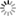##### Personal tools
You are here: Home AI and Algebra

# AI and Algebra

### - Overview

Algebra is one of the broad areas of mathematics, together with number theory, geometry and analysis. In its most general form, algebra is the study of mathematical symbols and the rules for manipulating these symbols; it is a unifying thread of almost all of mathematics. It includes everything from elementary equation solving to the study of abstractions such as groups, rings, and fields.

Algebra is divided into different sub-branches such as elementary algebra, advanced algebra, abstract algebra, linear algebra, and commutative algebra. The more basic parts of algebra are called elementary algebra; the more abstract parts are called abstract algebra or modern algebra.

Elementary algebra is generally considered to be essential for any study of mathematics, science, or engineering, as well as such applications as medicine and economics. Abstract algebra is a major area in advanced mathematics, studied primarily by professional mathematicians.

### - Elementary Algebra

In general, knowledge of algebra may be the foundation of mathematics. In addition to mathematical operations such as addition, subtraction, multiplication, and division, you need to know the following: Exponents, Radicals, Factorials, Summations, Scientific Notations.: Exponents, Radicals, Factorials, Summations, Scientific Notations.

### - Algebra Formulas

Algebra helps to solve mathematical equations and allows to derive unknown quantities such as bank interest, ratios, percentages. We can use variables in algebra to represent unknowns coupled in a way that rewrites the equation.

Algebraic formulas are used in our daily life to calculate the distance and volume of a container and, if needed, the sale price. Algebra is constructive in stating mathematical equations and relationships by using letters or other symbols that represent entities. The unknowns in the equations can be solved algebraically.

Some of the major topics under algebra include basics of algebra, exponentials, simplification of algebraic expressions, polynomials, quadratic equations, and more.

Please refer to the following for details:

### - Modern Algebra

In mathematics, more specifically, algebra, abstract algebra or modern algebra is the study of algebraic structures. Algebraic structures include algebras on groups, rings, fields, modules, vector spaces, lattices, and fields. The term abstract algebra was coined in the early 20th century to distinguish this field of study from earlier parts of algebra and, more specifically, from elementary algebra (the use of variables to represent numbers in computation and reasoning).

Algebraic structures and their associated homomorphisms constitute mathematical categories. Category theory is a formalism that allows a variety of structurally similar properties and structures to be expressed in a unified way.
Universal algebra is a related discipline that studies the types of algebraic structures as a single object. For example, the structure of a group is a single object in universal algebra called the diversity of the group.### - Linear Algebra

Artificial Intelligence is not a single subject it has sub-fields like Learning (Machine Learning & Deep Learning), Communication using NLP, Knowledge Representation & Reasoning, Problem Solving, Uncertain Knowledge & Reasoning.

Linear algebra is the foundation of machine learning, from the notations used to describe the algorithms, to the implementation of the algorithms itself.

Linear algebra is a branch of algebra that applies to both applied and pure mathematics. It handles linear mappings between vector spaces. It also involves the study of planes and lines. It is the study of systems of linear equations with transformation properties. It is used in almost all areas of mathematics. It deals with linear equations of linear functions and their representation in vector spaces and matrices. Important topics covered in Linear Algebra are as follows:

• System of linear equations
• Vector space
• Relation
• Matrices and Matrix Factorization
• Relationships and calculations

### - Linear Algebra You Need To Know for AI

Linear algebra is the primary mathematical computing tool in artificial intelligence and many other fields of science and engineering. Using this field, you need to know about the 4 main math objects and their properties:

• Scalar -  A single number (can be real or natural).
• Vectors  - An ordered list of numbers. Think of them as points in space, and each element represents a coordinate along an axis.
• Matrix - A two-dimensional array of numbers, where each number is identified by 2 indices.
• Tensor -   An N-dimensional array (N>2), arranged on a regular grid with N axes. Important in machine learning, deep learning and computer vision.
• Eigenvectors & Eigenvalues  -  Special vectors and their corresponding scalars. Learn about the importance and how to find them.
• Singular value decomposition  -  decomposes a matrix into 3 matrices. Learn about properties and applications.
• Principal Component Analysis (PCA)  -  understand meaning, properties and applications.
• Properties such as dot product, vector product, and Hadamard product are also useful.

### - Information Theory Concepts You Need to Know for AI

This is an important field that has made significant contributions to AI and Deep Learning. Think of it as an amalgamation of calculus, statistics, and probability.

• Entropy — also called Shannon Entropy. Used to measure the uncertainty in an experiment.
• Cross-Entropy — compares two probability distributions and tells us how similar they are.
• Kullback Leibler Divergence — another measure of how similar two probability distributions are.
• Viterbi Algorithm — widely used in Natural Language Processing (NLP) and Speech.
• Encoder-Decoder — used in Machine Translation RNNs and other models.

[More to come ...]

##### Document Actions